# MacLaurin formula

(diff) ← Older revision | Latest revision (diff) | Newer revision → (diff)

A particular case of the Taylor formula. Let a functionhavederivatives at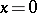. Then in some neighbourhoodof this pointcan be represented in the form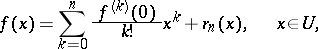where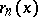, the-th order remainder term, can be represented in some form or other.

The term "MacLaurin formula" is also used for functions ofvariables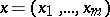. In this casein the MacLaurin formula is taken to be a multi-index,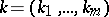(see MacLaurin series). The formula is named after C. MacLaurin.

For some expressions for the remainderand for estimates of it see Taylor formula.